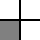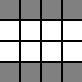Problem Statement

This problem contains HTML superscripts and images which will not display correctly for plugin users

Consider a finite two-dimensional grid where each cell is either occupied or empty. You are given two Strings, front and right, the frontal and right projections of the grid, respectively. The ith character of front indicates whether the ith column of grid is completely empty or not ("." for empty, lowercase "x" if the column contains at least one occupied cell). The ith character of right indicates whether the ith row of grid is completely empty or not.

Return a int[] containing exactly two elements. The first element is the minimum possible number of occupied cells on the grid, and the second element is the maximum possible number of occupied cells.

Definition

 Class: Projections Method: count Parameters: String, String Returns: int[] Method signature: int[] count(String front, String right) (be sure your method is public)

Constraints

-front will contain between 1 and 50 elements, inclusive.
-right will contain between 1 and 50 elements, inclusive.
-Each character in front and right will be "." or "x".
-Both front and right will contain at least one "x" character.

Examples

0)

 "x" "x"
Returns: {1, 1 }
 There is only one cell on the grid and it is obviously occupied.
1)

 "x." ".x"
Returns: {1, 1 }
 There is only one possible 2x2 grid with such projections (dark squares represent occupied cells):2)

 "xxxx" "x..x"
Returns: {4, 8 }
 One possible assignment with the minimum possible number of cells occupied is the following:The only possible assignment with the maximum possible number of cells occupied is the following:3)

 "x.x.x.x" "x.x.x.x"
Returns: {4, 16 }
4)

 "x...xx..x.xxx..x.xx." ".xx..xxx.xx."
Returns: {10, 70 }

Problem url:

http://www.topcoder.com/stat?c=problem_statement&pm=6041

Problem stats url:

http://www.topcoder.com/tc?module=ProblemDetail&rd=9820&pm=6041

Vovka

Testers:

PabloGilberto , brett1479 , vorthys , Olexiy

Simple Math